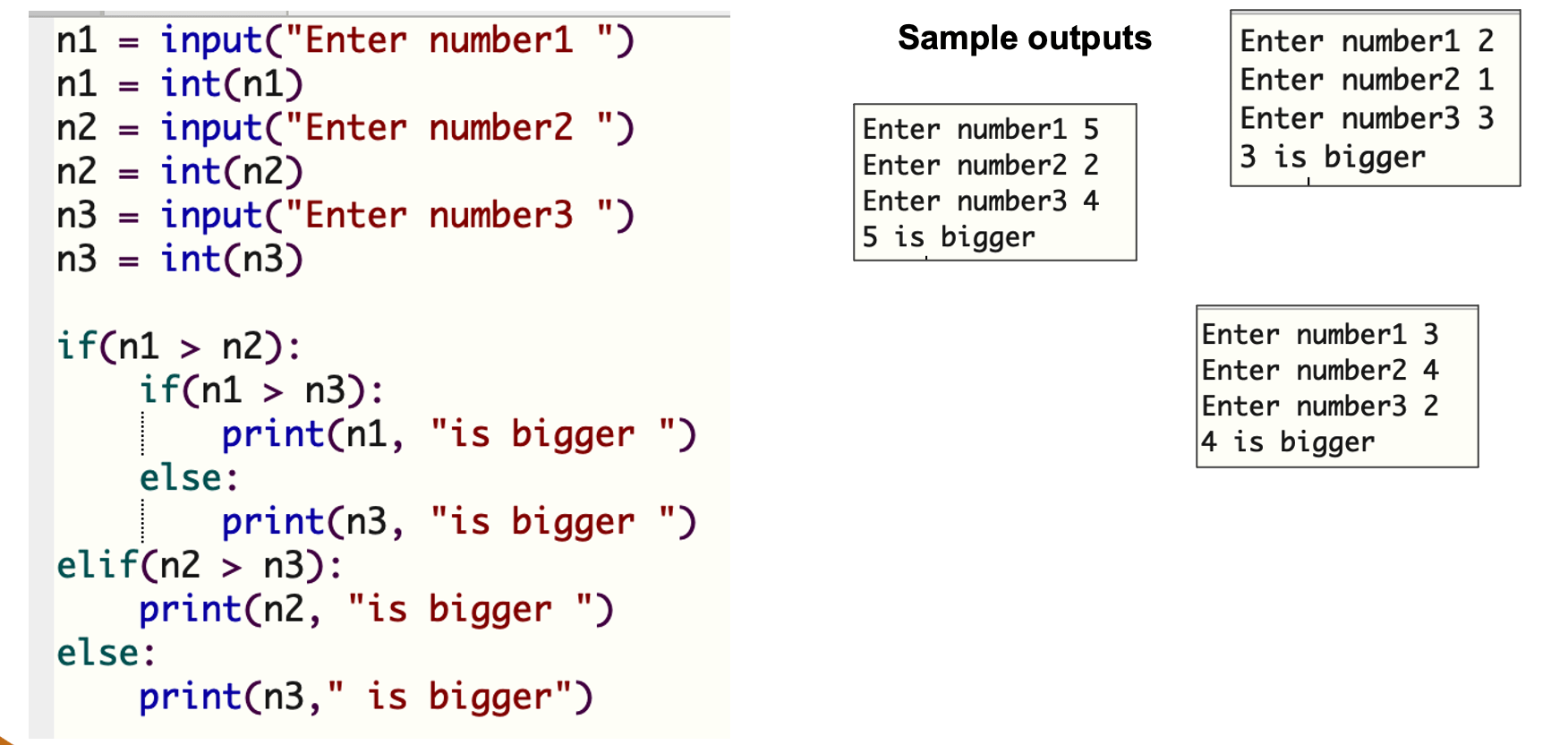# Nested Selections

## Nested if

There are other situations when you need to apply another condition even in case the first condition was True. In this case you need to use a normal if instead of process 1.

```	   if (condition-1):
if (condition-2):
process-1
else:
process-2
else:
process-3
```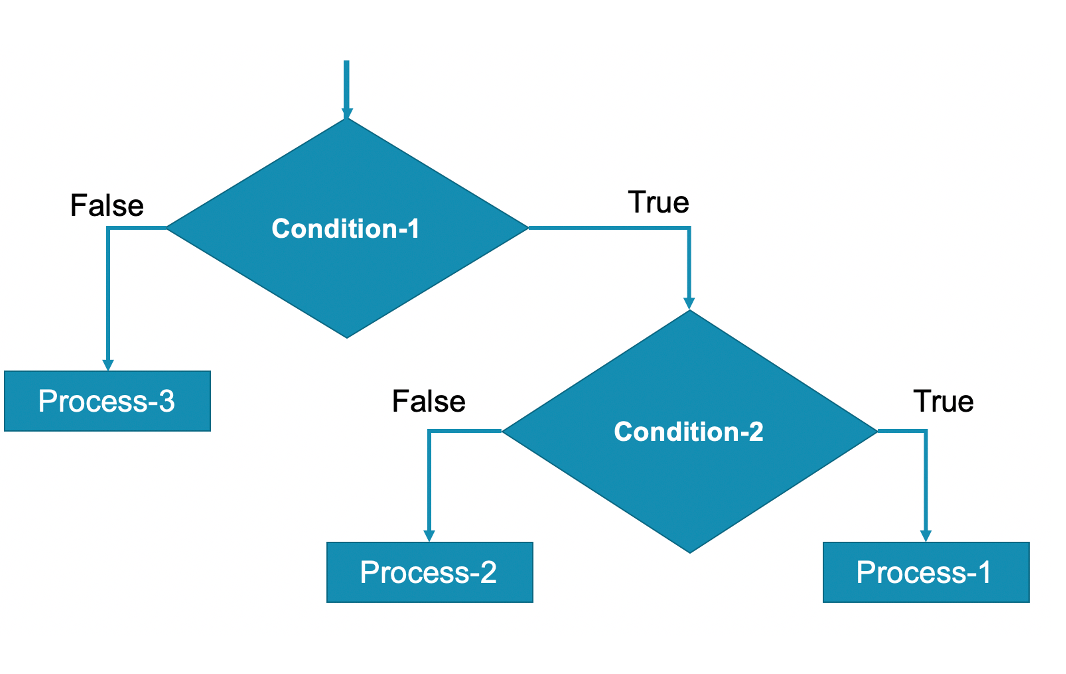### Example 1

Write a Python program to ask users to enter a number. The program determines and display given number is less than 1, single digit (1 – 9) or positive number that is more than one digit.

See below the code and its output: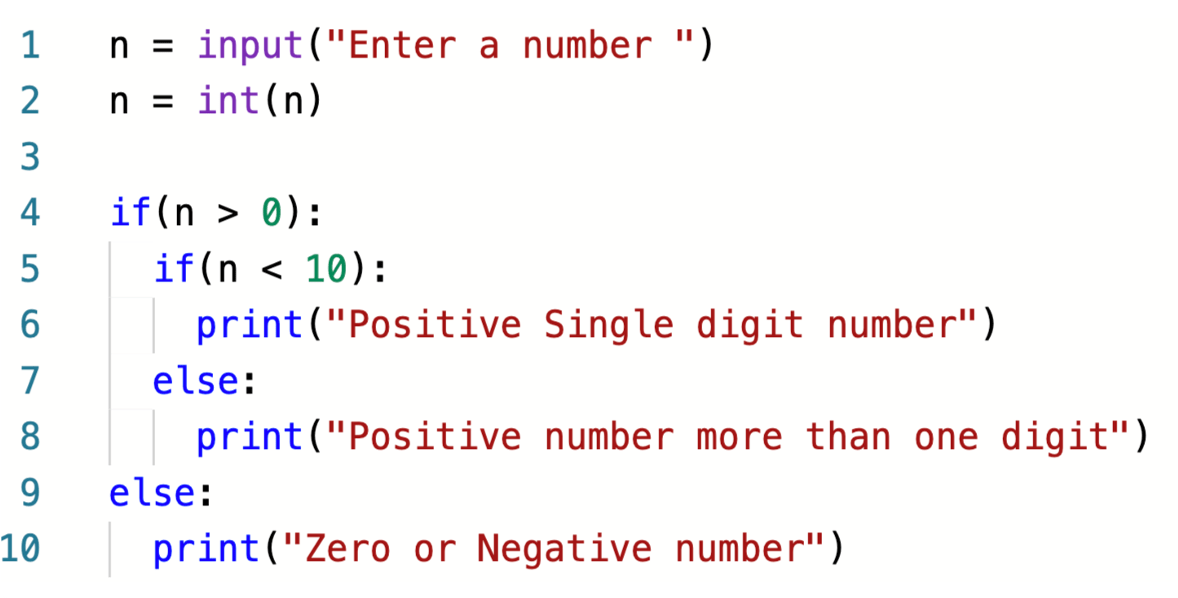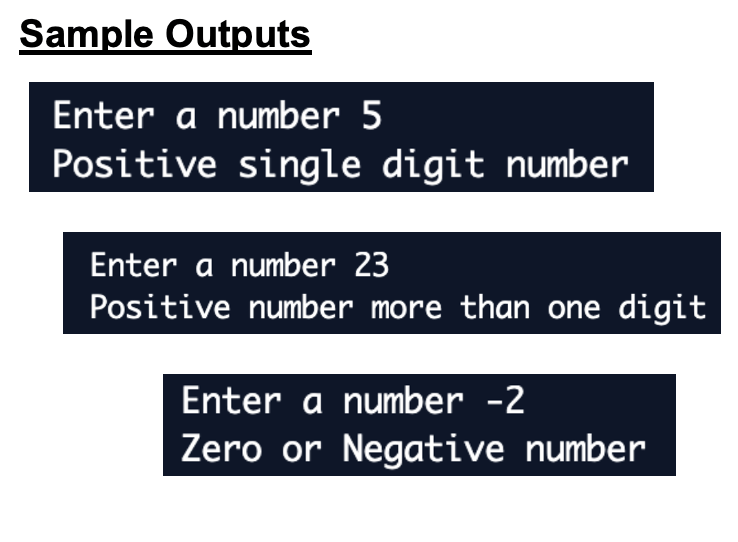## Nested else if

There are situations when you need to apply another condition in case the answer for the first condition is false. In this case we replace else with elif.

```	   if (condition-1):
process-1
elif (condition-2):
process-2
elif (condition-3):
process-3
else:
process-4
```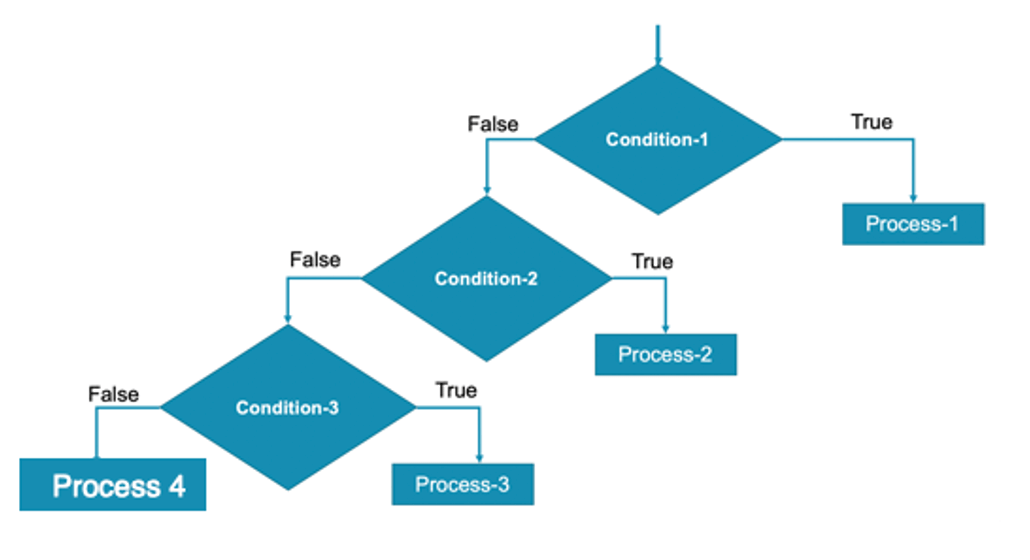### Example 2

Write a Python program to ask users to enter an age. The program determines and displays a message as shown in the below table.

 Age Message Up to 12 Child 13 to 19 Teenager 20 to 59 Adult 60 and beyond Senior

Here is the code and its output: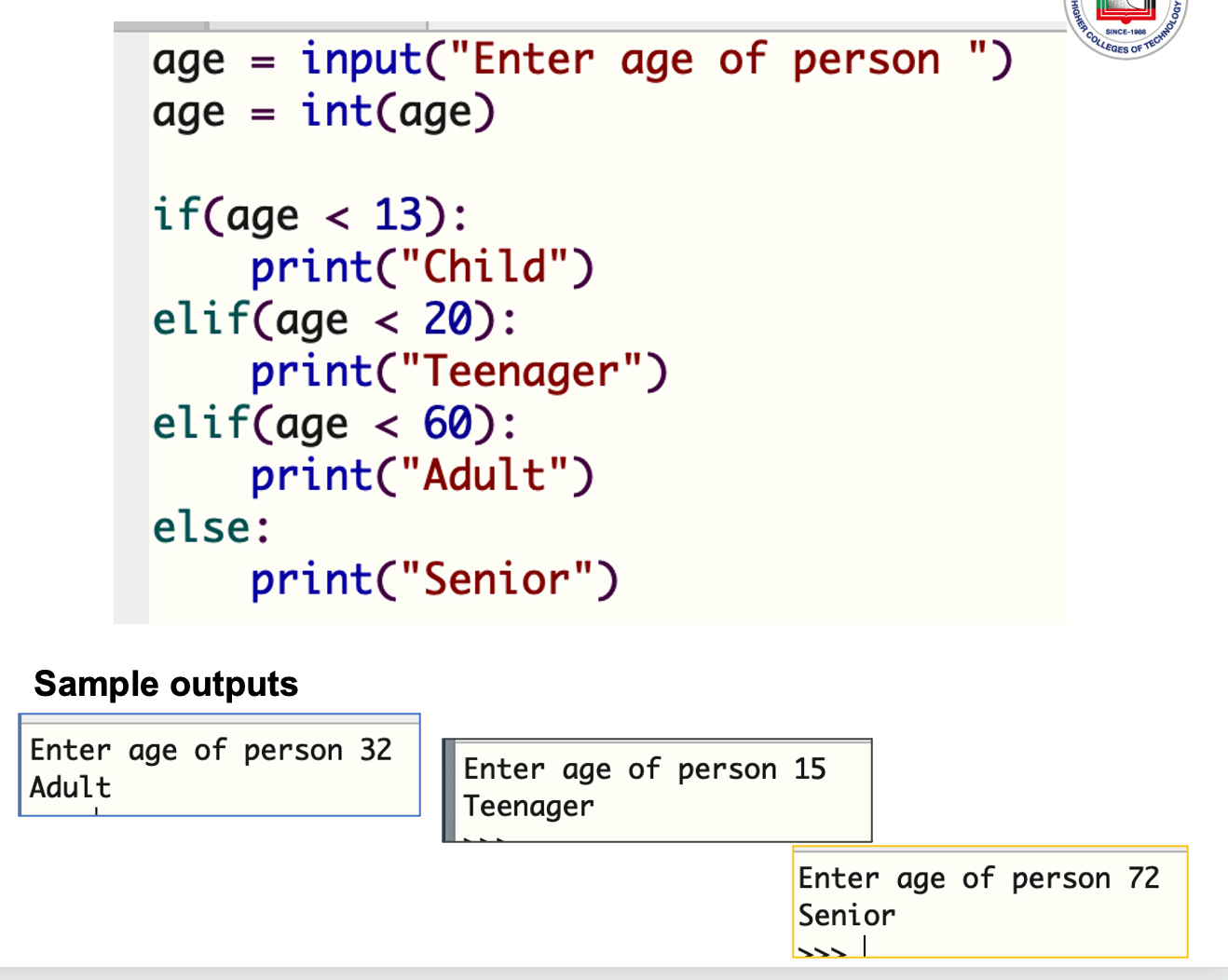## Nested if with nested else if

There are situations when you need to apply both nested if with nested else if.

```	   if (condition-1):
if (condition-2):
process-1
else:
process-2
elif (condition-3):
process-3
else:
process-4
```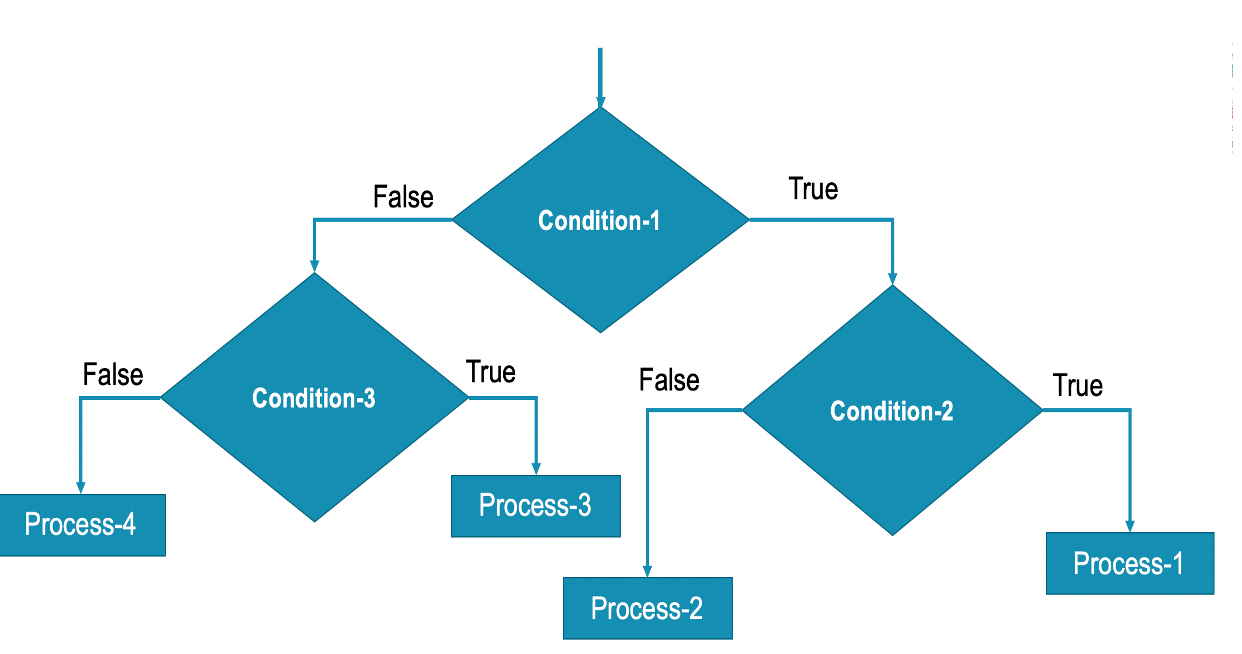### Example 3

Write a Python program to ask users to enter a three numbers. The program determines and display a the biggest number of the three.

Here is the code and its output: